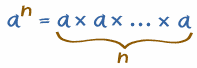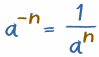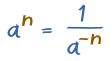# Using Exponents in Algebra

You might like to read the page on Exponents first.

## Whole Number Exponents

 The exponent "n" in an says how many times to use a in a multiplication:### Example: 53 = 5 × 5 × 5 = 125

• The "3" says to use 5 three times in a multiplication
• In words: 53 could be called "5 to the third power", "5 to the power 3" or simply "5 cubed"

## Negative ExponentsA negative exponent a−n is equal to 1/an (1 divided by the positive exponent)

### Example:

5−3 = 1/53 = 1/125 = 0.008

Also ... by changing the signs of the exponents we get:A positive exponent an is equal to 1/a−n (1 divided by the negative exponent)

So, we can move an expression between the top and bottom (numerator and denominator) of a fraction by simply changing the sign of the exponent.

Example: x−1 = 1/x (a simple reciprocal)

Example: 5−3 = 1/53 = 1/125 = 0.008

## Positive and Negative Together

Here is an example with positive and negative exponents:

### Example: 432-59-132

We can put the negative exponents at the bottom (and they become positive):

4332259

32 is 9:

439259

The 9s cancel out:

4325

43 is 4 × 4 × 4 = 64, and 25 is 2 × 2 × 2 × 2 × 2 = 32:

6432

Which simplifies to:

2

Done!